# Chapter 3 Pretest 1 After a body has

• Slides: 52Chapter 3 Pretest1. After a body has fallen freely from rest for 8. 0 s, its velocity is approximately: A) 40 m/s downward, B) 80 m/s downward, C) 120 m/s downward, D) 160 m/s downward.1. After a body has fallen freely from rest for 8. 0 s, its velocity is approximately: A) 40 m/s downward, B) 80 m/s downward, C) 120 m/s downward, D) 160 m/s downward.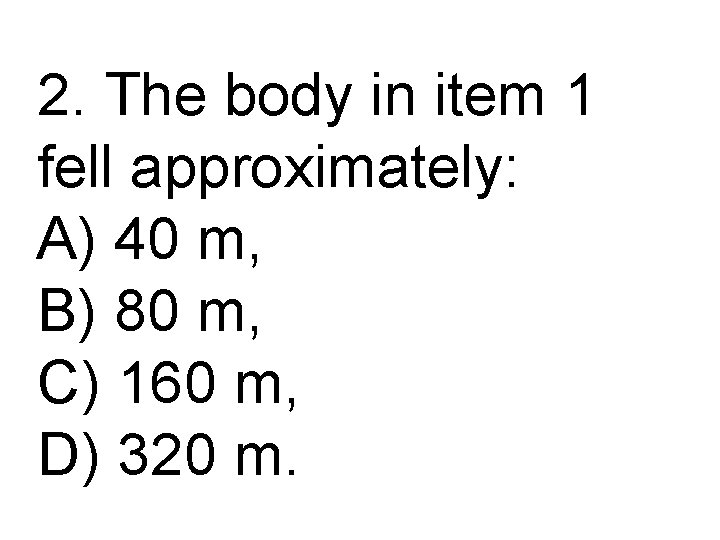2. The body in item 1 fell approximately: A) 40 m, B) 80 m, C) 160 m, D) 320 m.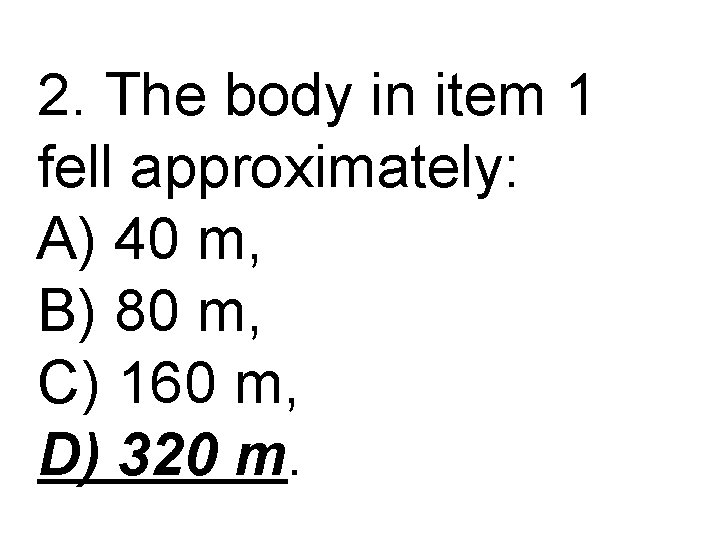2. The body in item 1 fell approximately: A) 40 m, B) 80 m, C) 160 m, D) 320 m.3. An object is accelerated from rest at the rate of 3. 0 m/s 2. After it has traveled 13. 5 m, its velocity is: A) 3. 0 m/s, B) 6. 0 m/s, C) 9. 0 m/s, D) 12. 0 m/s.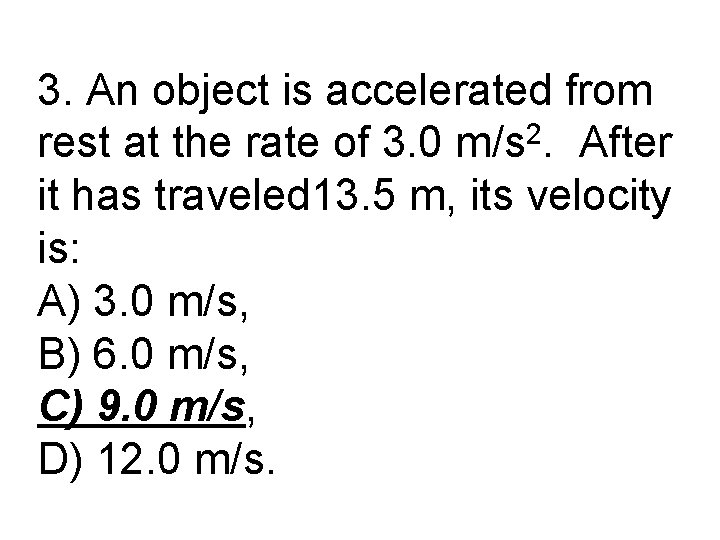3. An object is accelerated from rest at the rate of 3. 0 m/s 2. After it has traveled 13. 5 m, its velocity is: A) 3. 0 m/s, B) 6. 0 m/s, C) 9. 0 m/s, D) 12. 0 m/s.4. Which of the following is a physical quantity that has both magnitude and direction? a. vector b. scalar c. resultant d. frame of reference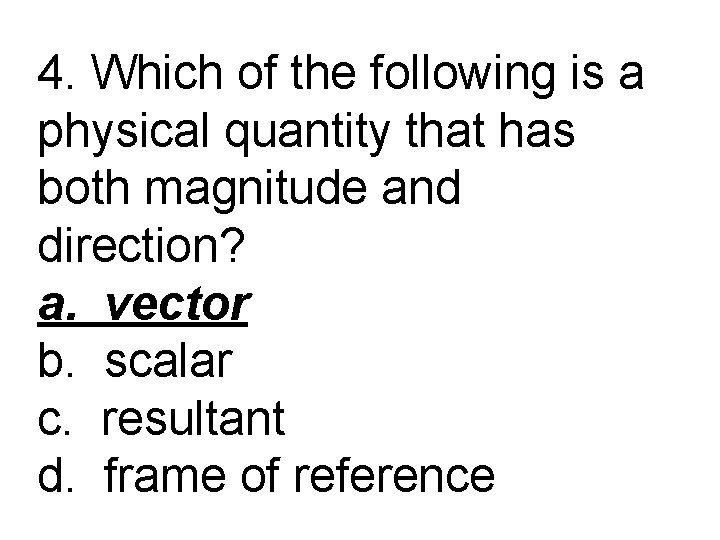4. Which of the following is a physical quantity that has both magnitude and direction? a. vector b. scalar c. resultant d. frame of reference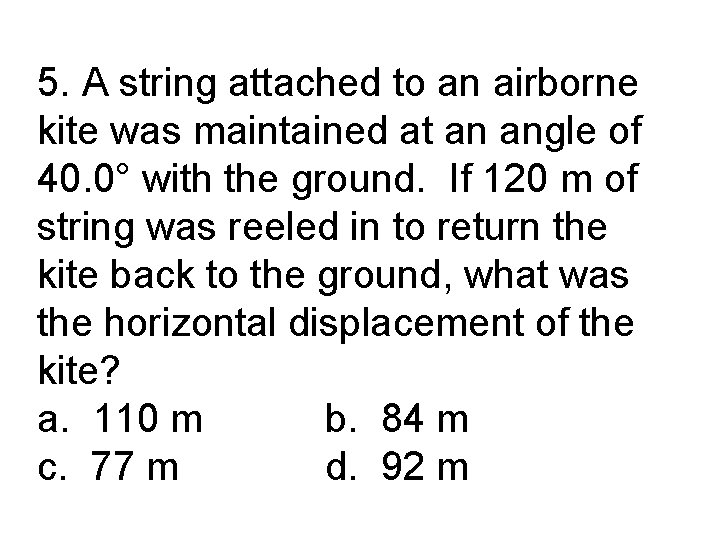5. A string attached to an airborne kite was maintained at an angle of 40. 0° with the ground. If 120 m of string was reeled in to return the kite back to the ground, what was the horizontal displacement of the kite? a. 110 m b. 84 m c. 77 m d. 92 m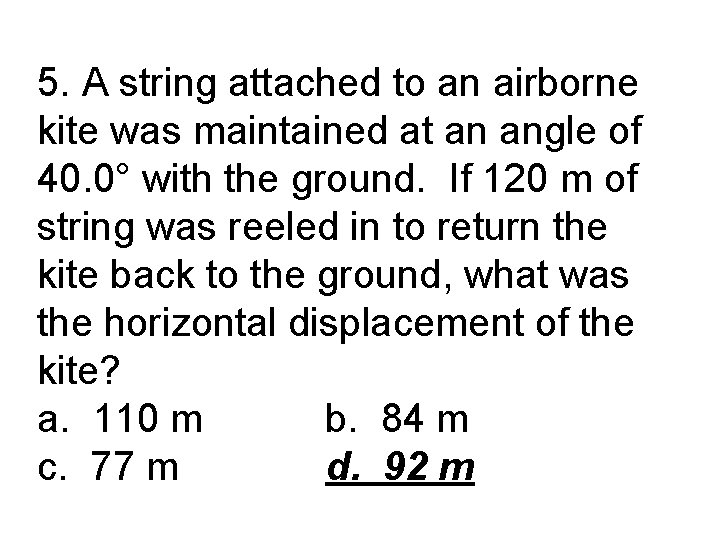5. A string attached to an airborne kite was maintained at an angle of 40. 0° with the ground. If 120 m of string was reeled in to return the kite back to the ground, what was the horizontal displacement of the kite? a. 110 m b. 84 m c. 77 m d. 92 m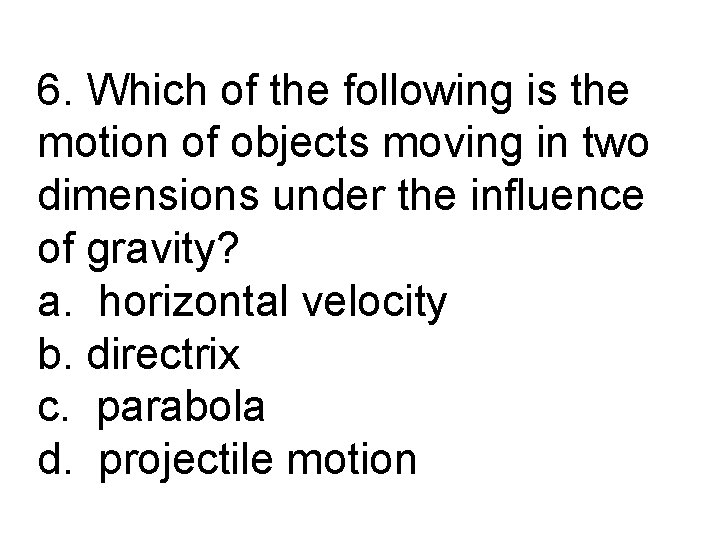6. Which of the following is the motion of objects moving in two dimensions under the influence of gravity? a. horizontal velocity b. directrix c. parabola d. projectile motion6. Which of the following is the motion of objects moving in two dimensions under the influence of gravity? a. horizontal velocity b. directrix c. parabola d. projectile motion7. What is the path of a projectile? a. a wavy line b. a parabola c. a hyperbola d. Projectiles do not follow a predictable path.7. What is the path of a projectile? a. a wavy line b. a parabola c. a hyperbola d. Projectiles do not follow a predictable path.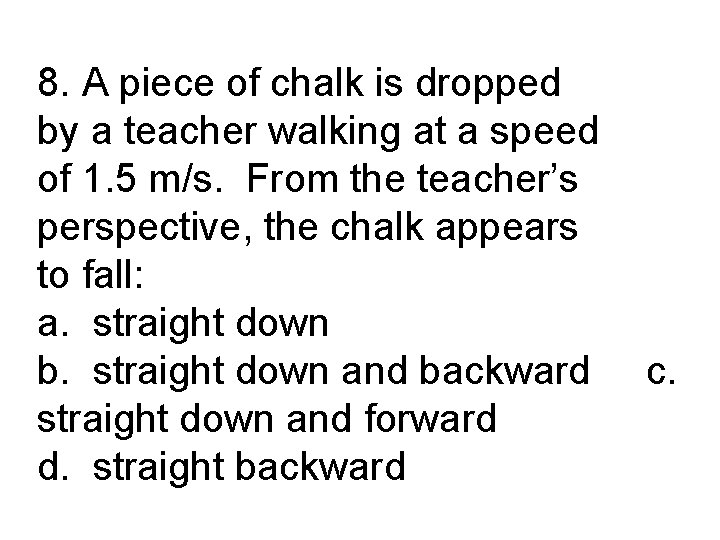8. A piece of chalk is dropped by a teacher walking at a speed of 1. 5 m/s. From the teacher’s perspective, the chalk appears to fall: a. straight down b. straight down and backward straight down and forward d. straight backward c.8. A piece of chalk is dropped by a teacher walking at a speed of 1. 5 m/s. From the teacher’s perspective, the chalk appears to fall: a. straight down b. straight down and backward straight down and forward d. straight backward c.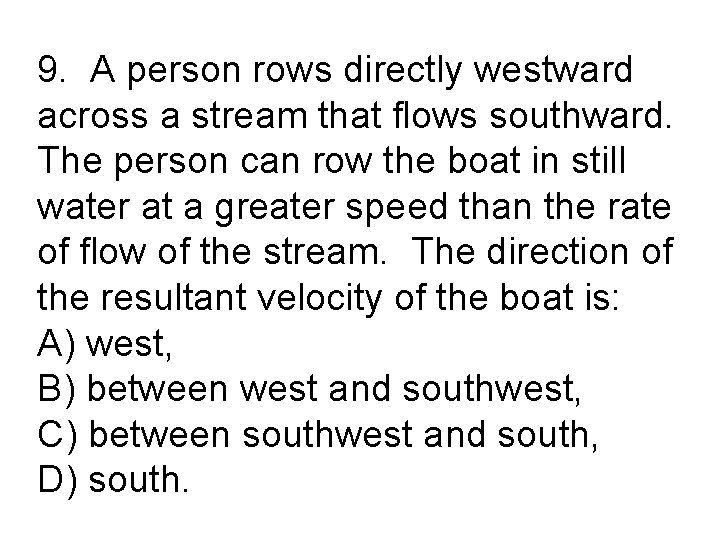9. A person rows directly westward across a stream that flows southward. The person can row the boat in still water at a greater speed than the rate of flow of the stream. The direction of the resultant velocity of the boat is: A) west, B) between west and southwest, C) between southwest and south, D) south.9. A person rows directly westward across a stream that flows southward. The person can row the boat in still water at a greater speed than the rate of flow of the stream. The direction of the resultant velocity of the boat is: A) west, B) between west and southwest, C) between southwest and south, D) south.10. If, in item 1, the person can row the boat at 6. 0 km/h and the stream flows at 3. 0 km/h, the magnitude of the resultant velocity is: A) less than 3. 0 km/h, B) between 3. 0 km/h and 6. 0 km/h, C) between 6. 0 km/h and 9. 0 km/h, D) greater than 9. 0 km/h.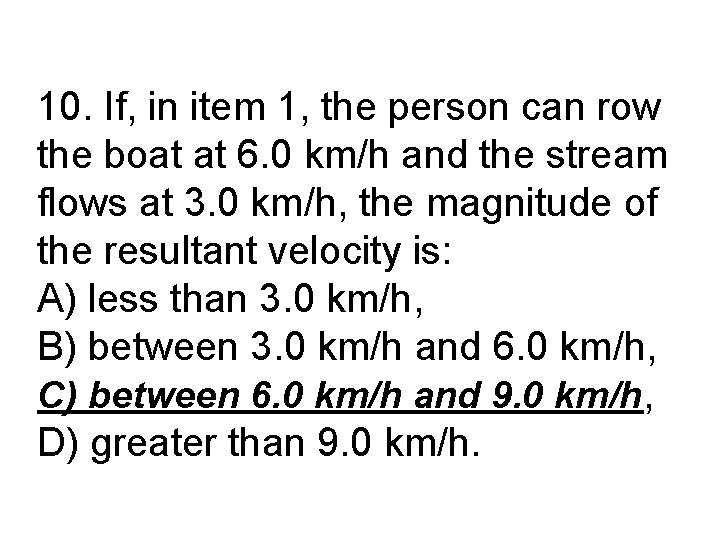10. If, in item 1, the person can row the boat at 6. 0 km/h and the stream flows at 3. 0 km/h, the magnitude of the resultant velocity is: A) less than 3. 0 km/h, B) between 3. 0 km/h and 6. 0 km/h, C) between 6. 0 km/h and 9. 0 km/h, D) greater than 9. 0 km/h.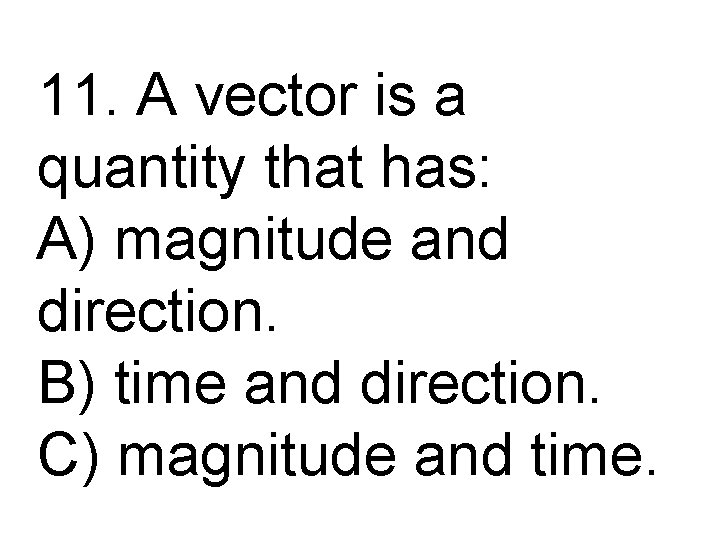11. A vector is a quantity that has: A) magnitude and direction. B) time and direction. C) magnitude and time.11. A vector is a quantity that has: A) magnitude and direction. B) time and direction. C) magnitude and time.12. The horizontal component of a projectile’s velocity is independent of: A) the vertical component of its velocity. B) time. C) the range of the projectile.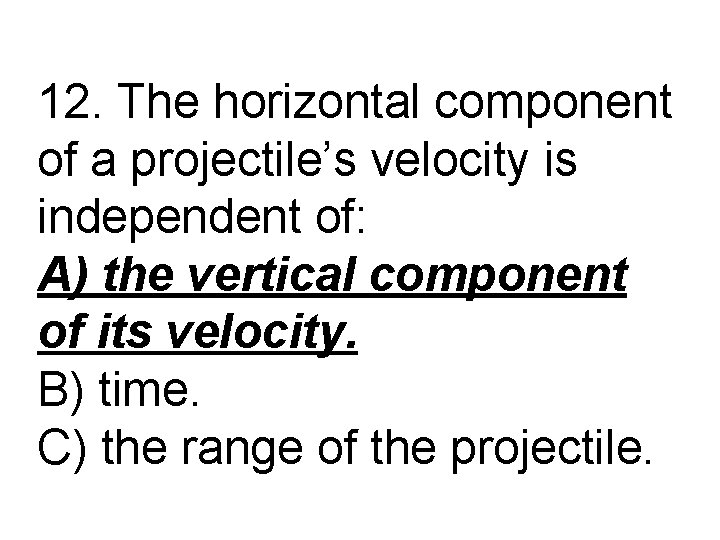12. The horizontal component of a projectile’s velocity is independent of: A) the vertical component of its velocity. B) time. C) the range of the projectile.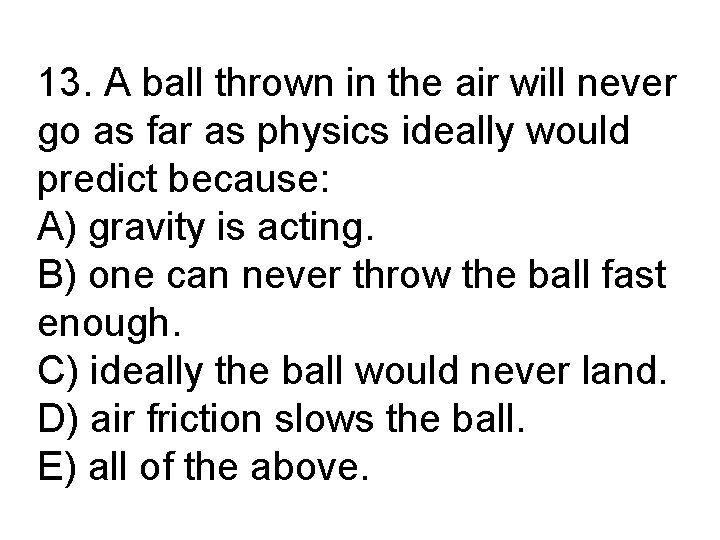13. A ball thrown in the air will never go as far as physics ideally would predict because: A) gravity is acting. B) one can never throw the ball fast enough. C) ideally the ball would never land. D) air friction slows the ball. E) all of the above.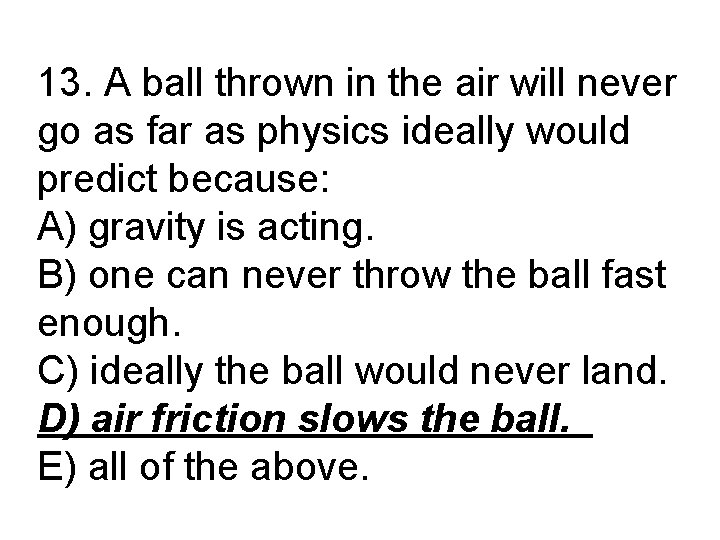13. A ball thrown in the air will never go as far as physics ideally would predict because: A) gravity is acting. B) one can never throw the ball fast enough. C) ideally the ball would never land. D) air friction slows the ball. E) all of the above.14. A ball is thrown straight upward at 20 m/s. Ideally (no air resistance), the ball will return to the thrower’s hand with a speed of: A) 0 m/s B) 40 m/s. C) 20 m/s. D) 10 m/s. E) There is not enough information to say.14. A ball is thrown straight upward at 20 m/s. Ideally (no air resistance), the ball will return to the thrower’s hand with a speed of: A) 0 m/s B) 40 m/s. C) 20 m/s. D) 10 m/s. E) There is not enough information to say.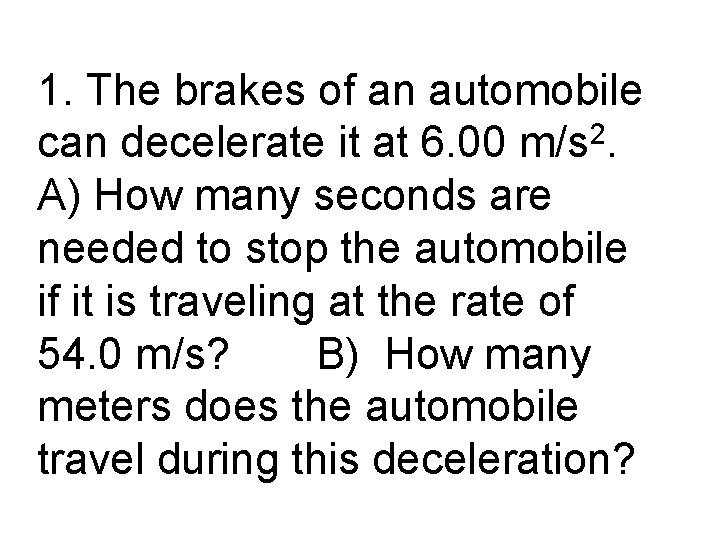1. The brakes of an automobile can decelerate it at 6. 00 m/s 2. A) How many seconds are needed to stop the automobile if it is traveling at the rate of 54. 0 m/s? B) How many meters does the automobile travel during this deceleration?(A) v = v 0 + at 0 = 54 + (-6)t t=9 s(B) x = v 0 t + ½ x = 54(9) + ½ 2 at 2 (-6)(9) x = 243 m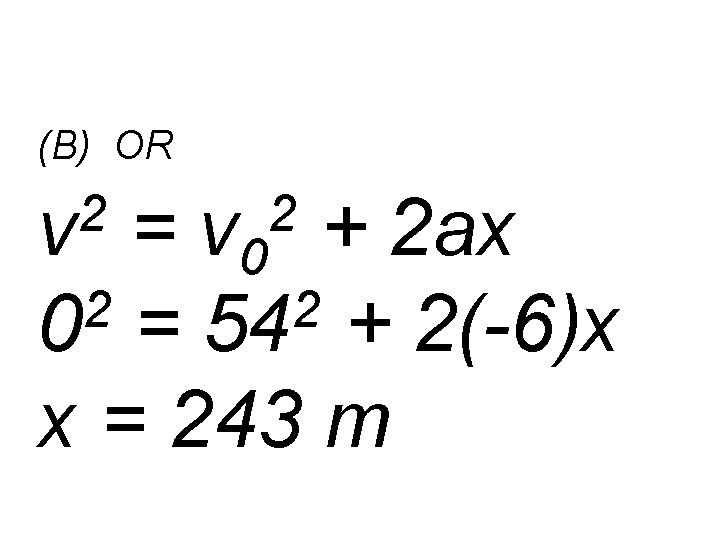(B) OR 2 v = v 0 + 2 ax 2 2 0 = 54 + 2(-6)x x = 243 m 2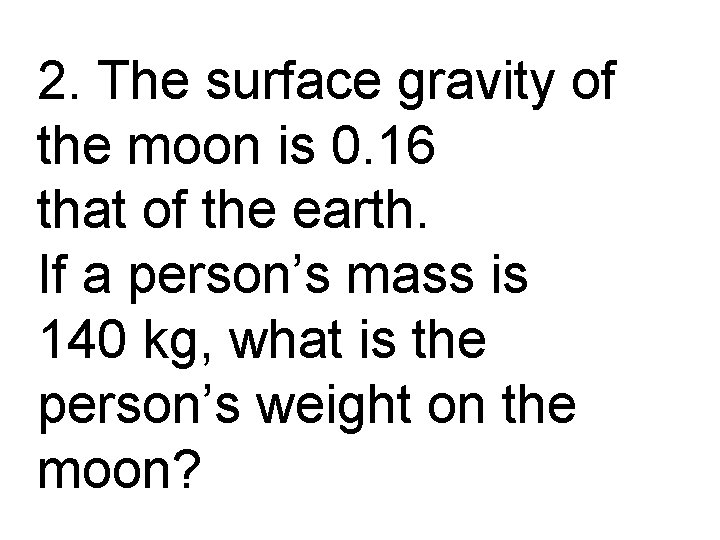2. The surface gravity of the moon is 0. 16 that of the earth. If a person’s mass is 140 kg, what is the person’s weight on the moon?140 x 10 x 0. 16 = 224 N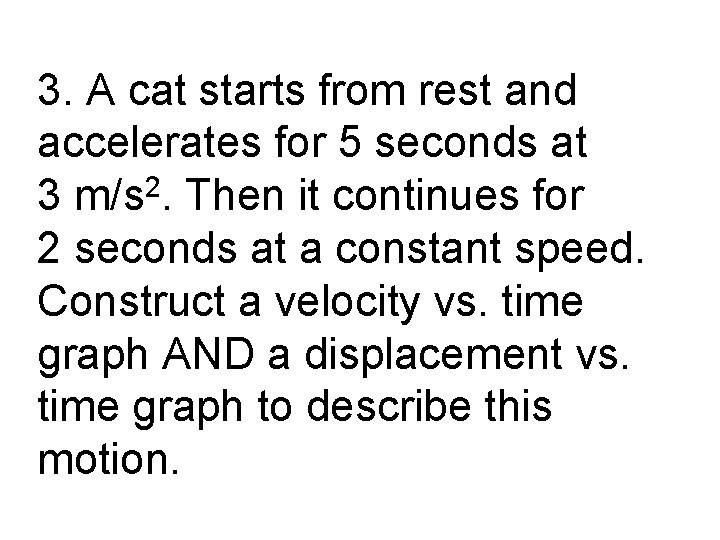3. A cat starts from rest and accelerates for 5 seconds at 3 m/s 2. Then it continues for 2 seconds at a constant speed. Construct a velocity vs. time graph AND a displacement vs. time graph to describe this motion.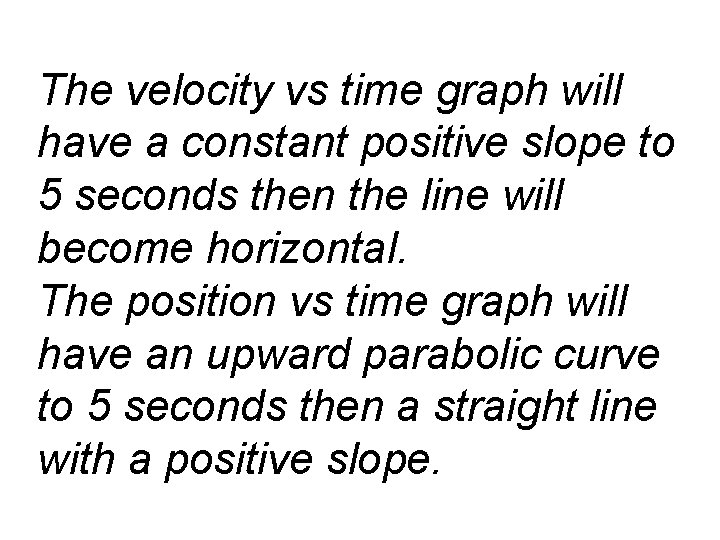The velocity vs time graph will have a constant positive slope to 5 seconds then the line will become horizontal. The position vs time graph will have an upward parabolic curve to 5 seconds then a straight line with a positive slope.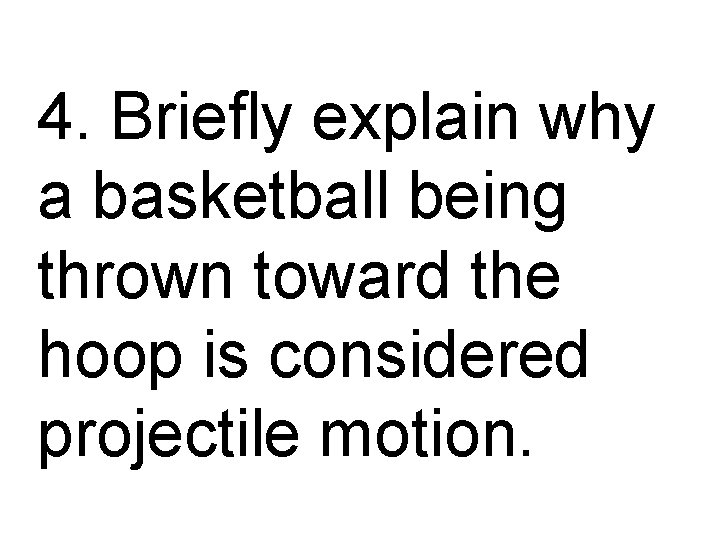4. Briefly explain why a basketball being thrown toward the hoop is considered projectile motion.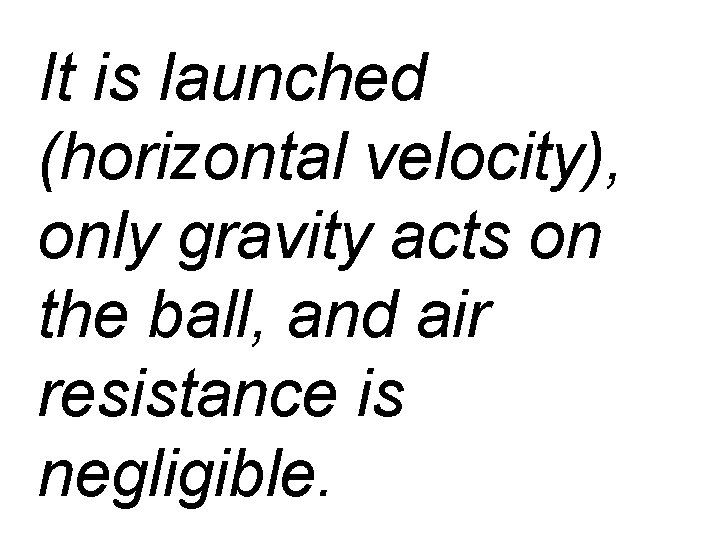It is launched (horizontal velocity), only gravity acts on the ball, and air resistance is negligible.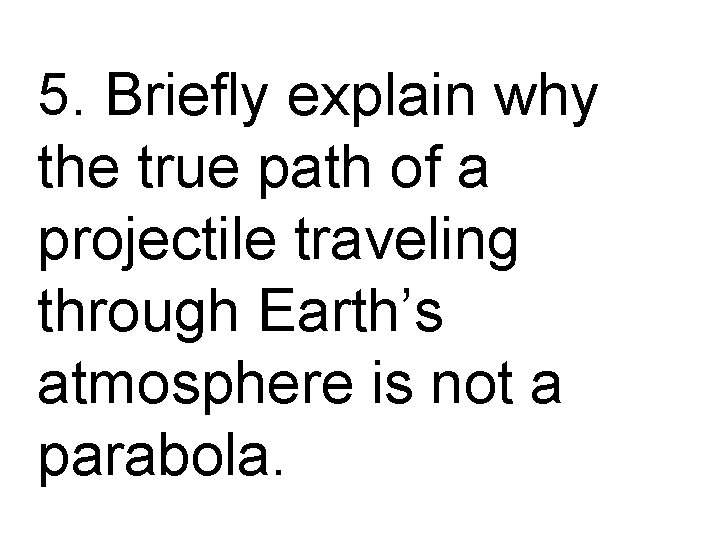5. Briefly explain why the true path of a projectile traveling through Earth’s atmosphere is not a parabola.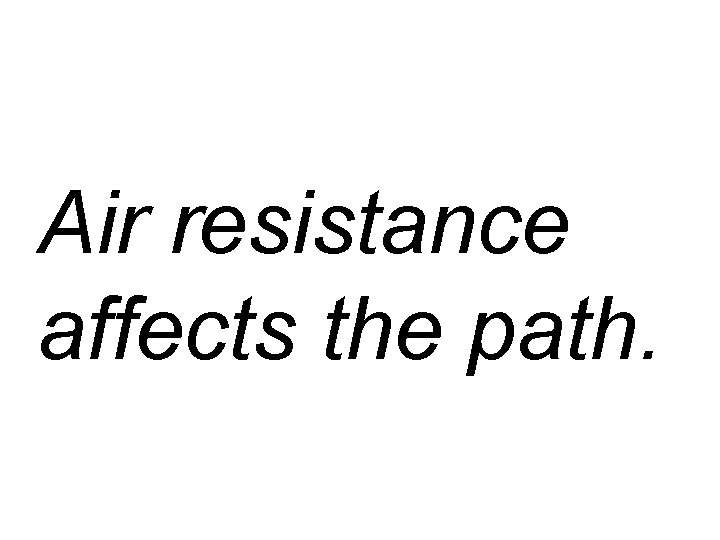Air resistance affects the path.6. On point 0, a force of 25. 0 N acts eastward and a force of 10. 0 N acts southward. Calculate the magnitude and direction of the resultant force.2 25 2 10 2 c + = c = 27 N tanq = 10/25 22° south of east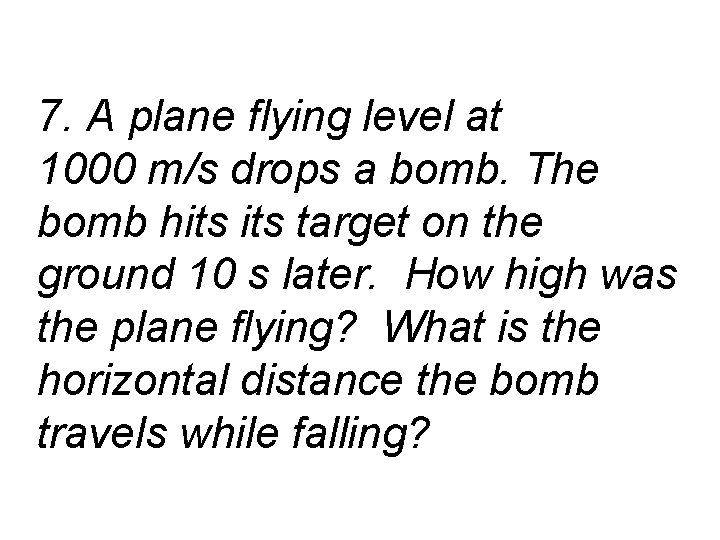7. A plane flying level at 1000 m/s drops a bomb. The bomb hits target on the ground 10 s later. How high was the plane flying? What is the horizontal distance the bomb travels while falling?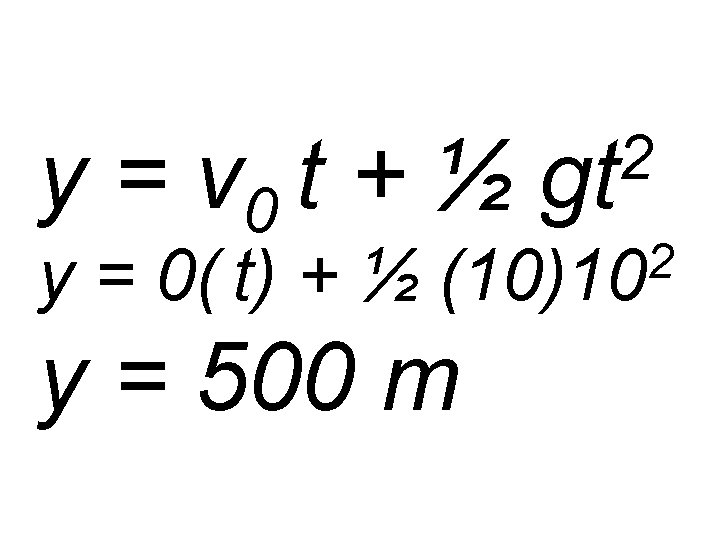y = v 0 t + ½ y = 0( t) + ½ 2 gt 2 (10)10 y = 500 m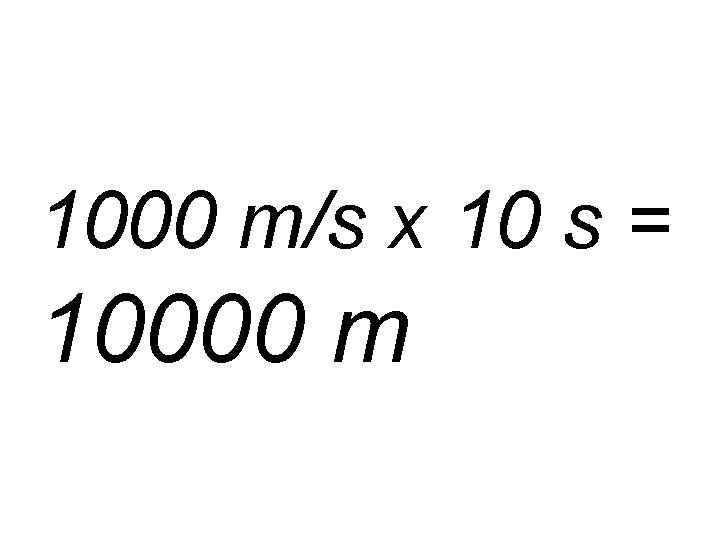1000 m/s x 10 s = 10000 m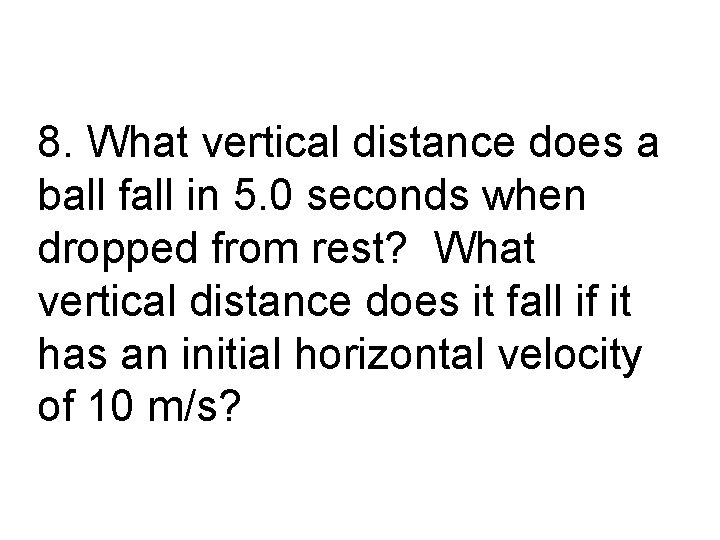8. What vertical distance does a ball fall in 5. 0 seconds when dropped from rest? What vertical distance does it fall if it has an initial horizontal velocity of 10 m/s?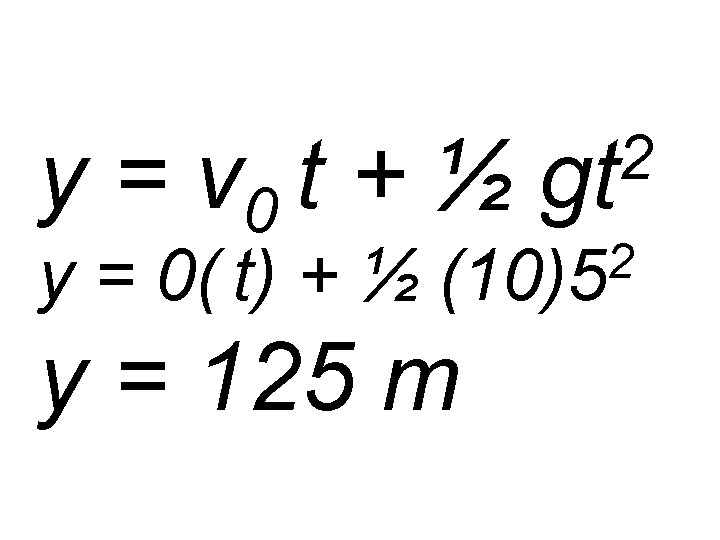y = v 0 t + ½ y = 0( t) + ½ 2 gt 2 (10)5 y = 125 m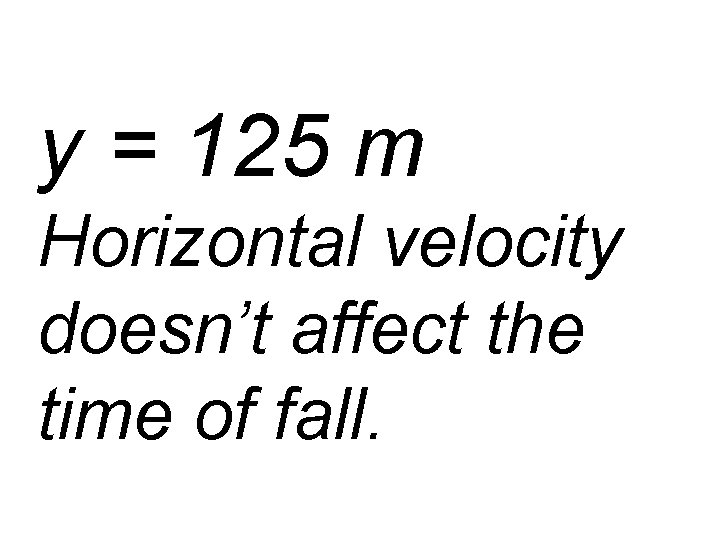y = 125 m Horizontal velocity doesn’t affect the time of fall.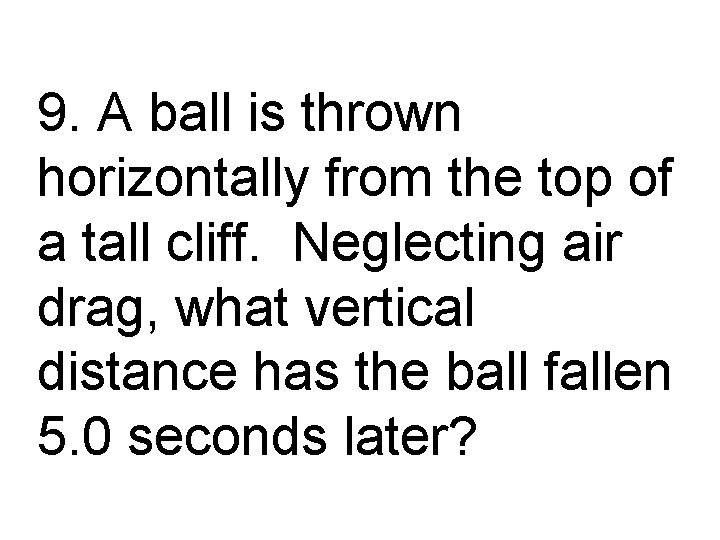9. A ball is thrown horizontally from the top of a tall cliff. Neglecting air drag, what vertical distance has the ball fallen 5. 0 seconds later?y = v 0 t + ½ y = 0( t) + ½ 2 gt 2 (10)5 y = 125 m